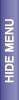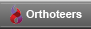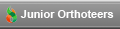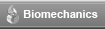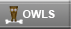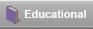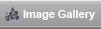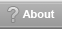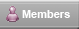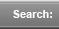# Choosing a Statistical Test

REVIEW OF AVAILABLE STATISTICAL TESTS

To select the right test, ask yourself two questions: What kind of data have you collected? What is your goal? Then refer to Table 37.1.

Table 37.1. Selecting a statistical test

 Goal Measurement (from Gaussian Population) Rank, Score, or Measurement (from Non- Gaussian Population) Binomial (Two Possible Outcomes) Survival Time Describe one group Mean, SD Median, interquartile range Proportion Kaplan Meier survival curve Compare one group to a hypothetical value One-sample t test Wilcoxon test Chi-square or Binomial test ** Compare two unpaired groups Unpaired t test Mann-Whitney test Fisher's test (chi-square for large samples) Log-rank test or Mantel-Haenszel* Compare two paired groups Paired t test Wilcoxon test McNemar's test Conditional proportional hazards regression* Compare three or more unmatched groups One-way ANOVA Kruskal-Wallis test Chi-square test Cox proportional hazard regression** Compare three or more matched groups Repeated-measures ANOVA Friedman test Cochrane Q** Conditional proportional hazards regression** Quantify association between two variables Pearson correlation Spearman correlation Contingency coefficients** Predict value from another measured variable Simple linear regression or Nonlinear regression Nonparametric regression** Simple logistic regression* Cox proportional hazard regression* Predict value from several measured or binomial variables Multiple linear regression* or Multiple nonlinear regression** Multiple logistic regression* Cox proportional hazard regression*

This is a preview of the site content. To view the full text for this site, you need to log in.
If you are having problems logging in, please refer to the login help page.

 © 2005-2007 Orthoteers.co.uk - last updated by Len Funk on 11 February 2005 Medical Merketing and SEO by Blue Medical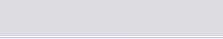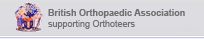Orthoteers is a non-profit educational resource. Click here for more details